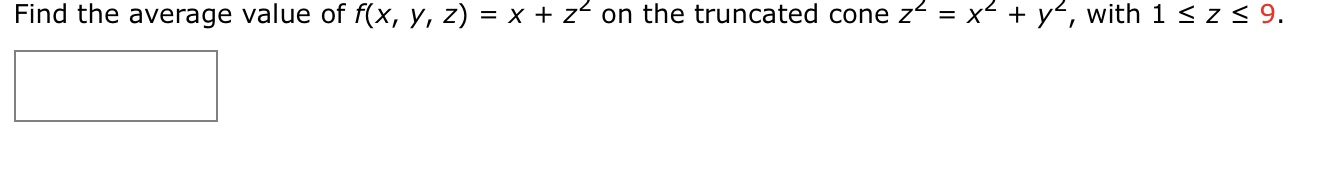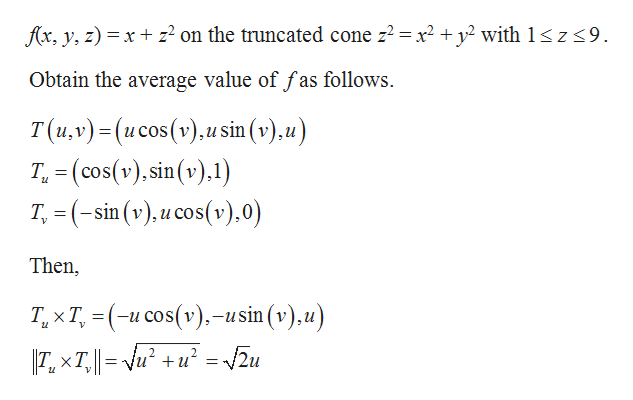# x z on the truncated cone z = x2+ y, with 1 < z < 9.Find the average value of f(x, y, z)

Question
32 viewshelp_outlineImage Transcriptionclosex z on the truncated cone z = x2+ y, with 1 < z < 9. Find the average value of f(x, y, z) fullscreen
check_circle

Step 1

From the given s...help_outlineImage Transcriptionclosefx, y, z) xz2 on the truncated cone z2 = x2 + y2 with 1< z <9 Obtain the average value of fas follows T(u,v)-(ucos(v).usin (v),u) T (cos(v),sin(v) Tsin (y).u cos(v).0) Then T, xT, (u cos(v)-usin (v),u) I7. xT,-vu +u =v2u fullscreen

### Want to see the full answer?

See Solution

#### Want to see this answer and more?

Solutions are written by subject experts who are available 24/7. Questions are typically answered within 1 hour.*

See Solution
*Response times may vary by subject and question.
Tagged in

### Math